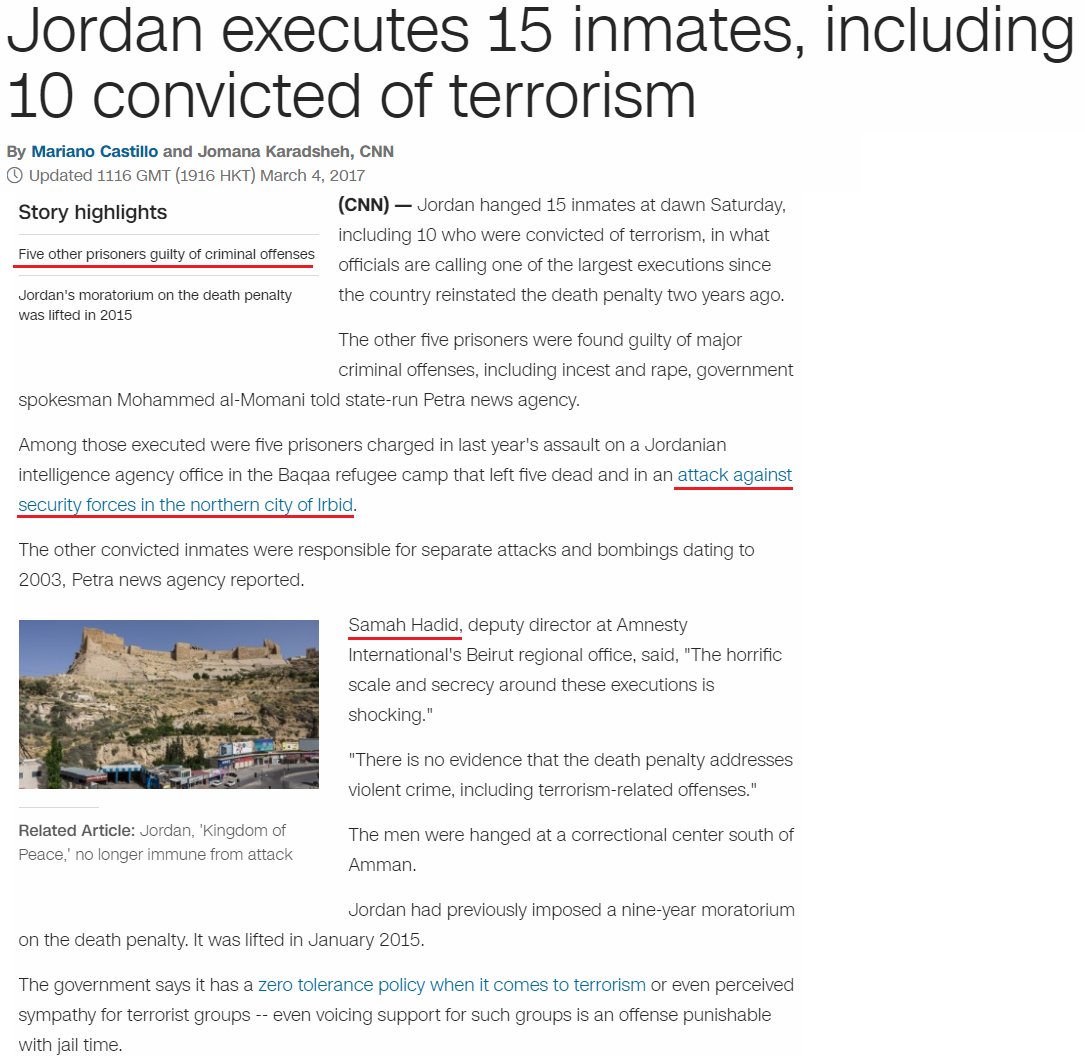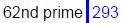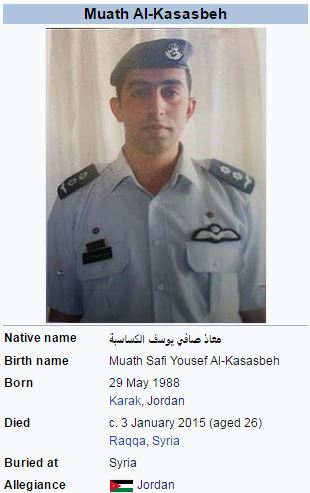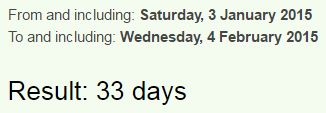CipherMar 4, 2017

CNN - Jordan executes 15 inmates, exactly 1 year after 'criminal plot linked to ISIS'

Link of the article - http://edition.cnn.com/2017/03/04/middleeast/jordan-inmates-execution/index.html

This was just released and we can always see all the connections clearly before they start updating the story, this one has some very interesting connecting to the previous executionsJordan executes 15 inmates = 17+12+9+23+26+13 + 22+3+22+24+6+7+22+8 + 18+13+14+26+7+22+8 = 322 (Reverse Ordinal) - calculator ignores the numbers, and this comes up to 322, the number of skull and bones and reflection of 223 (The Synagogue of Satan)

Jordan executes 15 inmates, including 10 convicted of terrorism = 8+3+9+5+8+4 + 4+3+4+6+6+7+4+8 + 9+4+5+8+7+4+8 + 9+4+6+6+6+5+9+4+2 + 6+3+4+5+9+6+7+4+5 + 3+3 + 7+4+9+9+3+9+9+8+5 = 293 (Reverse Reduced)
Among those executed were five prisoners charged in last year's assault on a Jordanian intelligence agency office in the Baqaa refugee camp that left five dead and in an attack against security forces in the northern city of Irbid.

The letters in blue take us to a story that was released on 3/3/2016, a date leaving 303 days in the year, and the title of the story has perfectly matching gematria with the story coming todayJordan foils criminal plot linked to ISIS in deadly raid = 8+3+9+5+8+4 + 3+3+9+6+8 + 6+9+9+5+9+4+8+6 + 2+6+3+7 + 6+9+4+7+4+5 + 7+3 + 9+8+9+8 + 9+4 + 5+4+8+5+6+2 + 9+8+9+5 = 293 (Reverse Reduced)Jordan = 10+15+18+4+1+14 = 62 (Ordinal)
Sixty two = 1+9+6+2+7 + 2+5+6 = 38 (Reduced)
ISIS = 9+10+9+10 = 38 (S Exception)
Today they executed 15 inmates - fifteen = 6+9+6+2+5+5+5 = 38 (Reduced)
Death = 4+5+1+20+8 = 38 (Ordinal), Killing = 2+9+3+3+9+5+7 = 38 (Reduced), Murder = 5+6+9+5+4+9 = 38 (Reverse Reduced)

Of course the execution is happening on a date with 44 numerology
3/4/2017 - (3) + (4) + (20) + (17) = 44 - Execution = 5+6+5+3+3+2+9+6+5 = 44 (Reduced)
3/4/2017 - 3+4 + 2+0+1+7 = 17 Kill = 2+9+3+3 = 17 (Reduced), Kill = 11+9+12+12 = 44 (Ordinal)
Fourth of March = 6+6+3+9+2+8 + 6+6 + 4+1+9+3+8 = 71 (Reduced)Note that this story is coming 366 days after the "criminal plot" in 2016, much like a full circle which is the reason they have some 227 coding in this story which connects to stuff from 2015In the story highlights they wrote - "Five other prisoners guilty of criminal offenses" = 6+9+4+5 + 6+2+8+5+9 + 7+9+9+1+6+5+5+9+1 + 7+3+9+3+2+7 + 6+6 + 3+9+9+4+9+5+1+3 + 6+6+6+5+5+1+5+1 = 227 (Reduced)

Last time they executed someone was on 2/4/2015
Fourth of February = 21+12+6+9+7+19 + 12+21 + 21+22+25+9+6+26+9+2 = 227 (Reverse Ordinal)
2 people were executed on that day
Ziad Khalaf Raja alKarbouly = 26+9+1+4 + 11+8+1+12+1+6 + 18+1+10+1 + 1+12+11+1+18+2+15+21+12+25 = 227 (Ordinal)
Sajida AlRishawi = 19+1+10+9+4+1 + 1+12+18+9+19+8+1+23+9 = 144 (Ordinal)
These 2 men supposedly killed a pilot Muath al-Kasasbeh on 3rd of January
Third of January = 2+8+9+9+4 + 6+6 + 1+1+5+3+1+9+7 = 71 (Reduced)
Seventy one = 144 (Ordinal)The 2 men were executed on the 33rd day after they killed Muath Al-KasasbehAl-Kasasbeh was killed on 1/3/2015 - (5) + (29) + 2+0+1+4 = 41 (13th prime number is 41)
The execution happened on a date with 41 numerology as well - 2/4/2015 (2) + (4) + (20) + (15) = 41
Jordan is located at 31°N
In February 2016 local media reported that a government committee had recommended the execution of 13 offenders, out of 80 investigated cases. A government spokesperson said that a decision was not yet taken41 - 13th prime number

All of this information I'm finding is from "Capital punishment in Jordan" Wikipedia page that I linked earlier, it has been updated about the same time as this story was rolling out today so they definitely helped me a lot here

The story from 3/3/2016 also has a lot of 13 connectionsAlso in the story coming today they are quoting someone called Samah HadidSamah Hadid = 1+1+4+1+8 + 8+1+4+9+4 = 41 (Reduced)
Samah Hadid = 90+1+30+1+8 + 8+1+4+9+4 = 156 (Jewish)
Thirty three = 20+8+9+18+20+25 + 20+8+18+5+5 = 156 (Ordinal)
In the beginning of the story they point out how these 15 inmates were executed at "dawn"
Dawn = 23+26+4+13 = 66 (Reverse Ordinal)
Thirty three = 2+8+9+9+2+7 + 2+8+9+5+5 = 66 (Reduced)
The men were hanged at a correctional center south of Amman.
south of Amman = 90+50+200+100+8 + 50+6 + 1+30+30+1+40 = 606 (Jewish)
Jordan hanged 15 inmates at dawn Saturday, including 10 who were convicted of terrorism, in what officials are calling one of the largest executions since the country reinstated the death penalty two years ago.

So they split them into group of 10 and group of 5 which is very interesting
Ten = 7+22+13 = 42 (Reverse Ordinal)
Five = 6+9+22+5 = 42 (Ordinal)
Amman = 1+13+13+1+14 = 42 (Ordinal)
dawn = 4+1+23+14 = 42 (Ordinal)